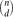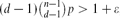Home

# Local Limit Theorems for the Giant Component of Random Hypergraphs†

## Abstract

Let Hd(n,p) signify a random d-uniform hypergraph with n vertices in which each of the$\binom{n}{d}$ possible edges is present with probability p=p(n) independently, and let Hd(n,m) denote a uniformly distributed d-uniform hypergraph with n vertices and m edges. We derive local limit theorems for the joint distribution of the number of vertices and the number of edges in the largest component of Hd(n,p) and Hd(n,m) in the regime$(d-1)\binom{n-1}{d-1}p>1+\varepsilon$ , resp. d(d−1)m/n>1+ϵ, where ϵ>0 is arbitrarily small but fixed as n → ∞. The proofs are based on a purely probabilistic approach.

## Footnotes

Hide All

An extended abstract version of this work appeared in the proceedings of RANDOM 2007, Vol. 4627 of Lecture Notes in Computer Science, Springer, pp. 341–352.

Hide All

# Local Limit Theorems for the Giant Component of Random Hypergraphs†

## Metrics

### Full text viewsFull text views reflects the number of PDF downloads, PDFs sent to Google Drive, Dropbox and Kindle and HTML full text views.

Total number of HTML views: 0
Total number of PDF views: 0 *Loading metrics...

### Abstract viewsAbstract views reflect the number of visits to the article landing page.

Total abstract views: 0 *Loading metrics...

* Views captured on Cambridge Core between <date>. This data will be updated every 24 hours.

Usage data cannot currently be displayed.